# Cases in Cost Management

## Quiz 23 : Peterson PotteryLooking for Management Homework Help?

## Quiz 23 :Peterson Pottery

Question Typea. What is your estimate of the 1983 income statement and balance sheet b. What is your estimate of Return on Assets in 1983 (Assume a 40% tax rate.) How is the company doing in 1983 For simplicity, you may assume that individual price and cost components have not changed between 1983 and 1985.
Free
Essay

a. Estimate of the income statement and balance sheet in the year 1983:
Following assumptions and facts are used for estimating income statement and balance sheet of MF, Inc. for the year 1983:
Assumptions and facts:
• Sales equals to $9,000,000 since 30,000 units were sold at the rate of$300 per unit of stoves.
• Out of total sales, let us assume 100% is cash sales. Therefore, cash amounts to $9,000,000 also. • The amount of$1,200,000 of materials can be considered as a part of inventory considering that there is no beginning level of inventory.
• Accounts receivable considered to be zero.
• Property equipment amounts to $12,000,000 which is determined by multiplying annual depreciation of$800,000 by the estimated life of 15 years.
• Accumulated depreciation assumed to be approximate $9,310,000 considering that property is in use since early seventies that means 1971. • Since Selling and shipping expenses were about two and a half times of what they were in the year 1983, therefore it comes out to be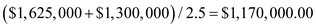. • Besides selling and shipping expenses, following expenses assumes to be incurred in producing 30,000 units of stove: General expenses:$1,500,000
Labor: $1,500,000 Rent:$550,000
Sales commission: $450,000 On the basis of above mentioned assumptions and facts, income statement and balance sheet of MF, Inc. for the year 1983 can be prepared as follows: Income Statement: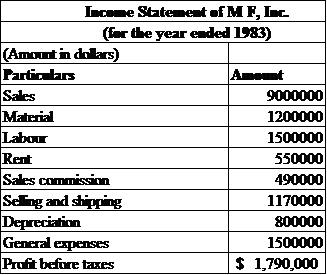Balance Sheet: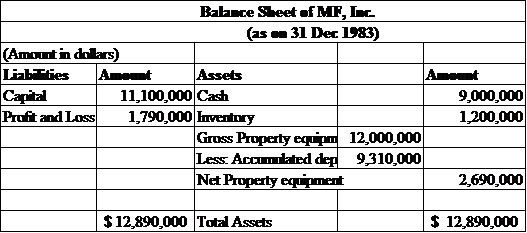(b) Estimate of Return on Asset (ROA) in 1983: Return on Asset (ROA): It is the percentage of net income that a company earns on its assets employed in the business. Net income is calculated by deducting taxes from Profit before taxes. Since Profit before taxes and total of assets employed are$1,790,000 and $12,890,000 respectively as per income statement and balance sheet. Therefore, Return on Asset (ROA) can be determined as follows: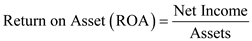Step 1: Calculation of Net Income: Net income can be calculated as follows: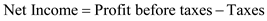Substitute:Hence, Net income is$1,074,000.
Step 2:
Calculation of Return on Asset (ROA):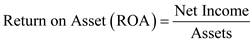Substitute:Hence, Return on Asset (ROA) of MF, Inc. for the year 1983 is 8%.
Conclusion:
Since higher the ROA is the better it is for a company. In this case, since it is 8% it means company has to put more efforts in terms of assets to earn more. It can also be said that company is not able to put its investment into profit since it is earning only $0.08 cents on each dollar of its investment. TagsTaking a closer look at cost allocation for manufacturing, selling and shipping expenses, what is your estimate of ovens profit and stoves profit for 1985 Free Essay Answer: Answer: Estimation of ovens profit and stove profits for the year 1985 considering cost allocation for manufacturing, selling and shipping expenses: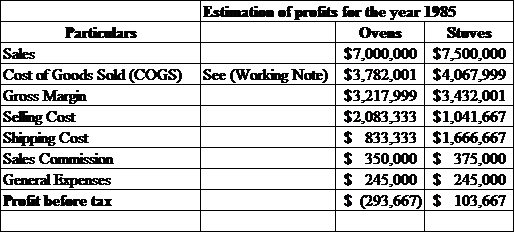In the above spreadsheet, cost of goods sold for Ovens and Stoves has been calculated by adding all the direct expenses. Gross margin is determined by deducting Cost of Goods sold from Sales and Profit before tax is determined by deducting all expenses like Selling, Shipping and Sales commission from gross margin. Thus, profits for stoves and ovens for the year 1985 are estimated as ($293,667) and $103,667. Working Note: Calculation of Cost of goods sold for Ovens and Stoves: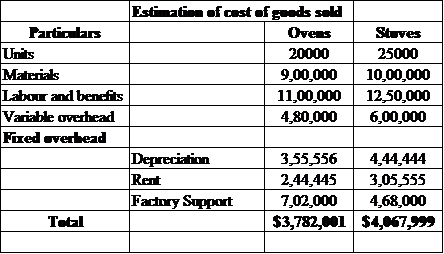TagsWhat is your estimate of the income statement for 1986 if only ovens were sold (30,000 units) Free Essay Answer: Answer: Estimate of the income statement for the year 1986 if only 30,000 units of ovens are sold: Following assumptions and facts are used for estimating income statement for the year 1986 when only 30,000 units of ovens are sold: Assumptions and facts: • There is no change in per unit cost and selling price. (Similar to 1985 figures) • Only 30,000 units are produced and sold of ovens. On the basis of above mentioned assumptions and facts, income statement for the year 1986 when only 30,000 units of ovens are sold can be prepared as follows: Income Statement: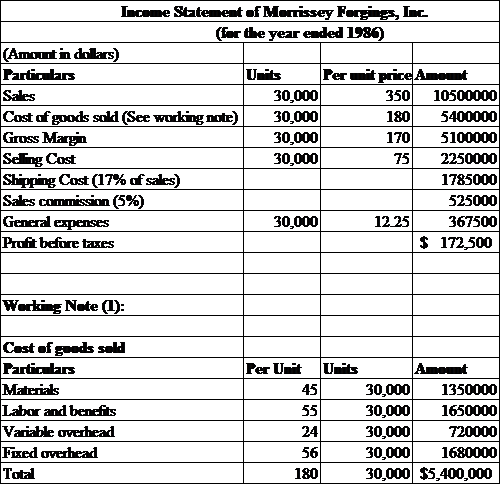Conclusion: On the basis of above workings, it can be estimated that in the year 1986 company can earn the profit of$172,500 by selling 30,000 units of ovens. (Keeping per unit costs as well as selling price constant as in the year 1985).

TagsHow much does it cost, on average, to ship a stove within the core area How much does it cost, on average, to ship a stove outside the core area
Essay
TagsHow much does it cost, on average, to generate a sales order for stoves in the core area (order getting costs) How much does it cost, on average, to generate a sales order for ovens outside the core area So what
Essay